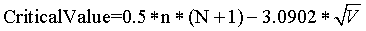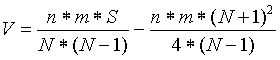';

## Title 40 Part 434 → Subpart H

### Title 40 Part 434 → Subpart H

e-CFR data is current as of December 9, 2019

 Title 40 → Chapter I → Subchapter N → Part 434 → Subpart H

Title 40: Protection of Environment
PART 434—COAL MINING POINT SOURCE CATEGORY BPT, BAT, BCT LIMITATIONS AND NEW SOURCE PERFORMANCE STANDARDS

## §434.80   Specialized definitions.

(a) The term brushing and grubbing area means the area where woody plant materials that would interfere with soil salvage operations have been removed or incorporated into the soil that is being salvaged.

(b) The term regraded area means the surface area of a coal mine that has been returned to required contour.

(c) The term sediment means undissolved organic and inorganic material transported or deposited by water.

(d) The term sediment yield means the sum of the soil losses from a surface minus deposition in macro-topographic depressions, at the toe of the hillslope, along field boundaries, or in terraces and channels sculpted into the hillslope.

(e) The term topsoil stockpiling area means the area outside the mined-out area where topsoil is temporarily stored for use in reclamation, including containment berms.

(f) The term western coal mining operation means a surface or underground coal mining operation located in the interior western United States, west of the 100th meridian west longitude, in an arid or semiarid environment with an average annual precipitation of 26.0 inches or less.

## §434.81   Applicability.

(a) This subpart applies to alkaline mine drainage at western coal mining operations from reclamation areas, brushing and grubbing areas, topsoil stockpiling areas, and regraded areas.

(b) This subpart applies to drainage at western coal mining operations from reclamation areas, brushing and grubbing areas, topsoil stockpiling areas, and regraded areas where the discharge, before any treatment, meets all the following requirements:

(1) pH is equal to or greater than 6.0;

(2) Dissolved iron concentration is less than 10 mg/L; and

(3) Net alkalinity is greater than zero.

(c) The effluent limitations in this subpart apply until the appropriate SMCRA authority has authorized bond release.

## §434.82   Effluent limitations attainable by the application of the best practicable control technology currently available (BPT).

Except as provided in 40 CFR 125.30 through 125.32, the following effluent limitations apply to mine drainage from applicable areas of western coal mining operations:

(a) The operator must submit a site-specific Sediment Control Plan to the permitting authority that is designed to prevent an increase in the average annual sediment yield from pre-mined, undisturbed conditions. The Sediment Control Plan must be approved by the permitting authority and be incorporated into the permit as an effluent limitation. The Sediment Control Plan must identify best management practices (BMPs) and also must describe design specifications, construction specifications, maintenance schedules, criteria for inspection, as well as expected performance and longevity of the best management practices.

(b) Using watershed models, the operator must demonstrate that implementation of the Sediment Control Plan will result in average annual sediment yields that will not be greater than the sediment yield levels from pre-mined, undisturbed conditions. The operator must use the same watershed model that was, or will be, used to acquire the SMCRA permit.

(c) The operator must design, implement, and maintain BMPs in the manner specified in the Sediment Control Plan.

## §434.83   Effluent limitations attainable by application of the best available technology economically achievable (BAT).

Except as provided in 40 CFR 125.30 through 125.32, any existing western coal mining operation with drainage subject to this subpart must meet the effluent limitations in §434.82.

## §434.85   New source performance standards (NSPS).

Any new source western coal mining operation with drainage subject to this subpart must meet the effluent limitations in §434.82.40v32(5546): Appendix A to Part 430

## Appendix A to Part 434—Alternate Storm Limitations for Acid or Ferruginous Mine Drainage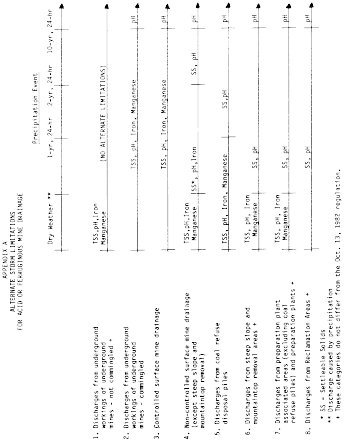# I. General Procedure Requirements

a. This appendix presents the procedures to be used for establishing effluent limitations for pre-existing discharges at coal remining operations, in accordance with the requirements set forth in subpart G; Coal Remining. The requirements specify that pollutant loadings of total iron, total manganese, total suspended solids, and net acidity in pre-existing discharges shall not exceed baseline pollutant loadings. The procedures described in this appendix shall be used for determining site-specific, baseline pollutant loadings, and for determining whether discharge loadings during coal remining operations have exceeded the baseline loading. Both a monthly (single-observation) procedure and an annual procedure shall be applied, as described below.

b. In order to sufficiently characterize pollutant loadings during baseline determination and during each annual monitoring period, it is required that at least one sample result be obtained per month for a period of 12 months.

c. Calculations described in this appendix must be applied to pollutant loadings. Each loading value is calculated as the product of a flow measurement and pollutant concentration taken on the same date at the same discharge sampling point, using standard units of flow and concentration (to be determined by the permitting authority). For example, flow may be measured in cubic feet per second, concentration in milligrams per liter, and the pollutant loading could be calculated in pounds per year.

d. Accommodating Data Below the Maximum Daily Limit at subpart C of this part. In the event that a pollutant concentration in the data used to determine baseline is lower than the daily maximum limitation established in subpart C of this part for active mine wastewater, the statistical procedures should not establish a baseline more stringent than the BPT and BAT effluent standards established in subpart C of this part. Therefore, if the total iron concentration in a baseline sample is below 7.0 mg/L, or the total manganese concentration is below 4.0 mg/L, the baseline sample concentration may be replaced with 7.0 mg/L and 4.0 mg/L, respectively, for the purposes of some of the statistical calculations in this appendix B. The substituted values should be used for all methods in this appendix B with the exception of the calculation of the interquartile range (R) in Method 1 for the annual trigger (Step 3), and in Method 2 for the single observation trigger (Step 3). The interquartile range (R) is the difference between the quartiles M−1 and M1; these values should be calculated using actual loadings (based on measured concentrations) when they are used to calculate R. This should be done in order to account for the full range of variability in the data.

# II. Procedure for Calculating and Applying a Single-Observation (Monthly) Trigger

Two alternative methods are provided for calculating a single-observation trigger. One method must be selected and applied by the permitting authority for any given remining permit.

## A. Method 1 for Calculating a Single Observation Trigger (L)

(1) Count the number of baseline observations taken for the pollutant of interest. Label this number n. In order to sufficiently characterize pollutant loadings during baseline determination and during each annual monitoring period, it is required that at least one sample result be obtained per month for a period of 12 months.

(2) Order all baseline loading observations from lowest to highest. Let the lowest number (minimum) be x(1), the next lowest be x(2), and so forth until the highest number (maximum) is x(n).

(3) If fewer than 17 baseline observations were obtained, then the single observation trigger (L) will equal the maximum of the baseline observations (x(n)).

(4) If at least 17 baseline observations were obtained, calculate the median (M) of all baseline observations:

Instructions for calculation of a median of n observations:

If n is odd, then M equals x(n/2 + 1/2).

For example, if there are 17 observations, then M = X(17/2 + 1/2) = x(9), the 9th highest observation.

If n is even, then M equals 0.5 * (x(n/2) + x(n/2 + 1)).

For example, if there are 18 observations, then M equals 0.5 multiplied by the sum of the 9th and 10th highest observations.

(a) Next, calculate M1 as the median of the subset of observations that range from the calculated M to the maximum x(n); that is, calculate the median of all x larger than or equal to M.

(b) Next, calculate M2 as the median of the subset of observations that range from the calculated M1 to x(n) ; that is, calculate the median of all x larger than or equal to M1.

(c) Next, calculate M3 as the median of the subset of observations that range from the calculated M2 to x(n) ; that is, calculate the median of all x larger than or equal to M2.

(d) Finally, calculate the single observation trigger (L) as the median of the subset of observations that range from the calculated M3 to x(n).

Note: When subsetting the data for each of steps 3a-3d, the subset should include all observations greater than or equal to the median calculated in the previous step. If the median calculated in the previous step is not an actual observation, it is not included in the new subset of observations. The new median value will then be calculated using the median procedure, based on whether the number of points in the subset is odd or even.

(5) Method for applying the single observation trigger (L) to determine when the baseline level has been exceeded

If two successive monthly monitoring observations both exceed L, immediately begin weekly monitoring for four weeks (four weekly samples).

(a) If three or fewer of the weekly observations exceed L, resume monthly monitoring

(b) If all four weekly observations exceed L, the baseline pollution loading has been exceeded.

## B. Method 2 for Calculating a Single Observation Trigger (L)

(1) Follow Method 1 above to obtain M1 (the third quartile, that is, the 75th percentile).

(2) Calculate M−1 as the median of the baseline data which are less than or equal to the sample median M.

(3) Calculate interquartile range, R = (M1 − M−1).

(4) Calculate the single observation trigger L as

L = M1 + 3 * R

(5) If two successive monthly monitoring observations both exceed L, immediately begin weekly monitoring for four weeks (four weekly samples).

(a) If three or fewer of the weekly observations exceed L, resume monthly monitoring

(b) If all four weekly observations exceed L, the baseline pollution loading has been exceeded.

# III. Procedure for Calculating and Applying an Annual Trigger

## A. Method 1 for Calculating and Applying an Annual Trigger (T)

(1) Calculate M and M1 of the baseline loading data as described above under Method 1 for the single observation trigger.

(2) Calculate M−1 as the median of the baseline data which are less than or equal to the sample median M.

(3) Calculate the interquartile range, R = (M1 − M−1).

(4) The annual trigger for baseline (Tb) is calculated as: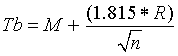(5) To compare baseline loading data to observations from the annual monitoring period, repeat steps 1-3 for the set of monitoring observations. Label the results of the calculations M′ and R′. Let m be the number of monitoring observations.

(6) The subtle trigger (Tm) of the monitoring data is calculated as: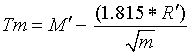## B. Method 2 for Calculating and Applying an Annual Trigger (T)

Method 2 applies the Wilcoxon-Mann-Whitney test to determine whether the median loading of the monitoring observations has exceeded the baseline median. No baseline value T is calculated.

### (1) Steps for Conducting the Wilcoxon-Mann-Whitney Test

(a) Let n be the number of baseline loading observations taken, and let m be the number of monitoring loading observations taken. In order to sufficiently characterize pollutant loadings during baseline determination and during each annual monitoring period, it is required that at least one sample result be obtained per month for a period of 12 months.

(b) Order the combined baseline and monitoring observations from smallest to largest.

(c) Assign a rank to each observation based on the assigned order: the smallest observation will have rank 1, the next smallest will have rank 2, and so forth, up to the highest observation, which will have rank n + m.

(1) If two or more observations are tied (have the same value), then the average rank for those observations should be used. For example, suppose the following four values are being ranked:

3, 4, 6, 4

Since 3 is the lowest of the four numbers, it would be assigned a rank of 1. The highest of the four numbers is 6, and would be assigned a rank of 4. The other two numbers are both 4. Rather than assign one a rank of 2 and the other a rank of 3, the average of 2 and 3 (i.e., 2.5) is given to both numbers.

(d) Sum all the assigned ranks of the n baseline observations, and let this sum be Sn.

(e) Obtain the critical value (C) from Table 1. When 12 monthly data are available for both baseline and monitoring (i.e., n = 12 and m = 12), the critical value C is 99.

### (2) Example Calculations for the Wilcoxon-Mann-Whitney Test

 BASELINE DATA 8.0 9.0 9.0 10.0 12.0 15.0 17.0 18.0 21.0 23.0 28.0 30.0 MONITORING DATA 9.0 10.0 11.0 12.0 13.0 14.0 16.0 18.0 20.0 24.0 29.0 31.0 BASELINE RANKS 1.0 3.0 3.0 5.5 8.5 12.0 14.0 15.5 18.0 19.0 21.0 23.0 MONITORING RANKS 3.0 5.5 7.0 8.5 10.0 11.0 13.0 15.5 17.0 20.0 22.0 24.0

Sum of Ranks for Baseline is Sn = 143.5, critical value is Cn, m = 99.

### (3) Critical Values for the Wilcoxon-Mann-Whitney Test

(a) When n and m are less than 21, use Table 1.

In order to find the appropriate critical value, match column with correct n (number of baseline observations) to row with correct m (number of monitoring observations).*

Table 1—Critical Values (C) of the Wilcoxon-Mann-Whitney Test

(for a one-sided test at the 0.001 significance level)

n
m
10

11

12

13

14

15

16

17

18

19

20

10667993109125142160179199220243
11688296112128145164183204225248
12708499115131149168188209231253
137387102118135153172192214236259
147589104121138157176197218241265
157791107124142161180201223246270
167994110127145164185206228251276
178196113130149168189211233257281
188399116134152172193215238262287
1985101119137156176197220243268293
2088104121140160180202224248273299

(b) When n or m is greater than 20 and there are few ties, calculate an approximate critical value using the following formula and round the result to the next larger integer. Let N = n + m.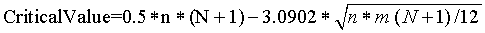For example, this calculation provides a result of 295.76 for n = m = 20, and a result of 96.476 for n = m = 12. Rounding up produces approximate critical values of 296 and 97.

(c) When n or m is greater than 20 and there are many ties, calculate an approximate critical value using the following formula and round the result to the next larger integer. Let S be the sum of the squares of the ranks or average ranks of all N observations. Let N = n + m.